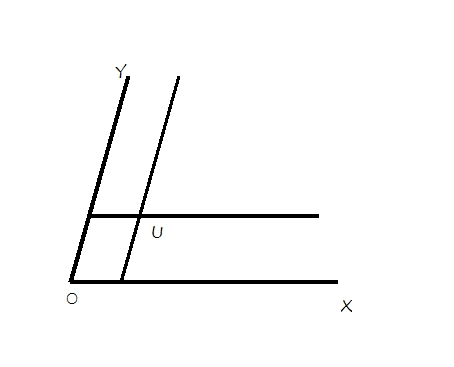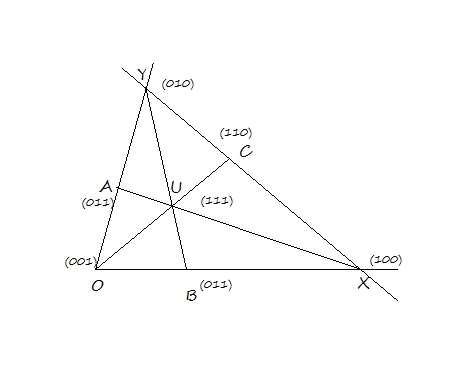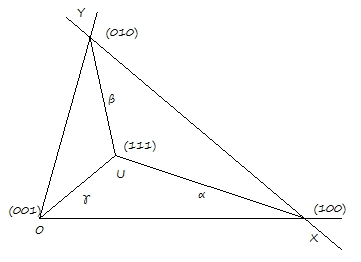# ijspace.org

 The Ubiquitous z-Axis 15th July 2015               The problem we have faced in developing a physical description in the discrete measurement space or j-space, is the precise determination of the origin due to the infinitesimal capacity of the macroscopic observer ObsM.  It is reflected in the value of the fine-structure constant.  Since the absolute values are not available, we need to find comparative methods to analyze a given system.  The two-state system is one of the consequences.  An example of a two-state system is the concept of "spin" in Quantum Mechanics, described by the Pauli matrices.  (We will discuss them within the context of Hamilton's Anharmonic Coordinates, a little later.)     The prerequisites to establish a two-state system, is the definition of the 0j using VT-symmetry, a symmetry dependent upon ObsM's capacity.  As we have discussed earlier this definition is equivalent to ¬∈ = ∈.  We are allowed to measure the continuum and the space, represented by axes OX and OY respectively, as shown in Fig-1.  Both OX and OY can extend up to infinity.  The definition of infinity will be different for OX and OY axes.  However the macroscopic observer is burdened with entropy.  Hence the measurements up to respective infinities will never be completed.      We define a unit point U, (1,1), whose projections on OX and OY,  represent the unit measurements in continuum and space respectively.  We keep in mind that OX and OY do not represent the axes in Euclidean space.  The objective is to define an arbitrary point P in terms of U in X-Y plane as shown.Figure-1: The definition of unit-point U in j-space.       We can account for the entropy inherent in the j-space by not allowing the observer to make measurements up to infinity on either OX or OY axes.  We connect the points on OX and OY axes where ObsM  resources are ineffective due to entropy and no further information can be measured.  Had there been no entropy, the observer would have continued up to infinity and the result would have been a true PE1 measurement.  Thus triangle OXY as shown in Figure-2 represents a PE<1 measurement space.  We are interested in developing a physical description within ΔOXY.   For ObsM it will represent PE1j space.Figure-2: The description of the measurement space for ObsM.         The unit-point U is now assigned a value (1,1,1).  Therefore the origin O in X-Y space is replaced by the unit point U.  The vertex represented by O is now taken as one of the vertices of the ΔOXY.   Note that the progress along OX and OY is limited by XY representing observer's upper limit and as a consequence the measured infinite for ObsM  is projected on to O.  Thus UO represents the traditional z-axis used to describe the physical measurements of a two-state system.  The vertices X, Y and O are represented by (100), (010), and (001) respectively.Figure-3: Anharmonic-Coordinate representation of the measurement space with respect to unit point U.     The lines UX, UY, and UZ can be extended to meet OY, OX, and XY at points A, B and C.  A geometrical net can be constructed by connecting B and C and extending it until it meets OY, connecting A and B until it meets XY, and connecting A and C until it meets OX.  A geometrical net from the elementary triangle ΔOXY originating from the unit-point U can be developed.  The nodes O, X, Y, and U are known as "cardinal points" of the net.  The points lying on the net are called "rational points".  The points lying outside the net, are called "irrational points".  The objective as explained earlier, is to construct or express an arbitrary point P in terms of the cardinal points.      Let us now consider the ΔOXY, and define UX, UY and UO as vectors having length α, β, γ and following the relationship that UX = -XU, UY = -YU, UO = -OU as shown in Figure-4. Please note that we have provided the points connected to U, directional properties of vectors.Figure-4: Directional properties of the net associated with the unit point U.   Given vectors UX, UY, and UO ( α,  β,  γ ), it is possible to find scalars l, m, n such that (Figure-3), if OB/BX = m/n;     XC/CY = l/m;     YA/AO = n/l, and OB/BX × XC/CY × YA/AO = 1, then lα + mβ + nγ = 0.     The values of the scalars l, m, and n must be non zero.  Further they must be either integers or rational numbers.  The ΔOXY with OUC, XUA, and YUB can be considered the primary net of point U (1, 1, 1). It has the following properties: (i) if l + m+ n = 0  then the net drops to a line.   (ii) if either of l, m, n is zero the net collapses to point U. (iii) The line [l, m, n] = [1, 1, 1] is called the unit line for an arbitrary point (α, β, γ).  For unit point (1, 1, 1) the unit line [1, 1, 1] is denoted by Λ.   The relationship between a unit line corresponding to the unit point, is that of a polar and its pole, such that the condition α + β + γ = 0, is satisfied.     We will discuss the description of an arbitrary point in ΔOXY next.  We should note that the construction being described here is analogous to the definition of "constructibility" from a unit length using compass and straight-edge in field theory.  Similar constraints of extensions of a field being rational numbers, are used to form the group of extensions which eventually lead to Galois theory to find solutions of higher order polynomials.  The irrational numbers are not part of such groups as they are not constructible.  In j-space we are forming similar structures in two-state system by constructing geometric nets around unit-point U, with projections of U on OX and OY axes as unit lengths.  The structures which are not constructible or do not lie on the geometric net, are not considered PE1j measurements in discrete measurement space or j-space.  For details on Geometric Nets and Anharmonic Coordinates, please refer to the works by Hamilton and Hime.Information on www.ijspace.org is licensed under a Creative Commons Attribution 4.0 International License.